# Polygon Problems

Examples of a regular polygon (polygon). Polygon is a closed part of the plane bounded by a broken line. Points that define a polygon are the polygon vertices. The number of vertices, sides, and interior angles in a polygon is the same, and this number specifies the name of the polygon: triangle, quadrangle, pentagon, etc.

#### Number of problems found: 107

• Diagonals in diamondIn the rhombus is given a = 160 cm, alpha = 60 degrees. Calculate the length of the diagonals.
• Sum of inner angles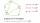Prove that the sum of all inner angles of any convex n-angle equals (n-2) . 180 degrees.
• Pentagon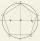Within a regular pentagon ABCDE point P is such that the triangle is equilateral ABP. How big is the angle BCP? Make a sketch.
• Hexagon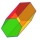Calculate the surface of a regular hexagonal prism whose base edge a = 12cm and side edge b = 3 dm.
• OctagonWe have a square with side 84 cm. We cut the corners to make his octagon. What will be the side of the octagon?
• DecagonCalculate the area and circumference of the regular decagon when its radius of a circle circumscribing is R = 1m
• Hexagon ACalculate area of regular hexagon inscribed in circle with radius r=9 cm.
• Angles of a hexagon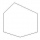Find the interior angles of a hexagon if the sizes of the angles form an arithmetic sequence and the smallest angle is 70°.
• Four sides of trapezoidTrapezoid is given by length of four sides: 40.5 42.5 52.8 35.0. Calculate its area.
• n-gon IIWhat is the side length of the regular 5-gon circumscribed circle of radius 11 cm?
• n-gonWhat is the side length of the regular 5-gon inscribed in a circle of radius 12 cm?
• Each with each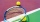Five pupils from 3A class played table tennis. How many matches did they play with each other?
• DiagonalsCalculate the length of the diagonals of the rhombus if its side is long 5 and one of its internal angle is 80°.
• Hexagon ABCDEFIn the regular hexagon ABCDEF, the diagonal AE has a length 8cm. Calculate the circumference and the hexagon area.
• AnnulusTwo concentric circles with radii 1 and 9 surround the annular circle. This ring is inscribed with n circles that do not overlap. Determine the highest possible value of n.
• Hexagon 5The distance of parallel sides of regular hexagonal is 61 cm. Calculate the length of the radius of the circle described to this hexagon.
• Regular 5-gonCalculate the area of the regular pentagon with side 7 cm.
• Circle and hexagon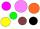Calculate the radius of a circle whose circumference is 8.4 cm longer than the circumference of the inscribed regular hexagon.
• Octahedron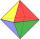All walls of regular octahedron are identical equilateral triangles. ABCDEF octahedron edges have a length d = 6 cm. Calculate the surface area and volume of this octahedron.
• Nine-gonCalculate the perimeter of a regular nonagon (9-gon) inscribed in a circle with a radius 13 cm.

Do you have an interesting mathematical word problem that you can't solve it? Submit a math problem, and we can try to solve it.

We will send a solution to your e-mail address. Solved examples are also published here. Please enter the e-mail correctly and check whether you don't have a full mailbox.

Please do not submit problems from current active competitions such as Mathematical Olympiad, correspondence seminars etc...